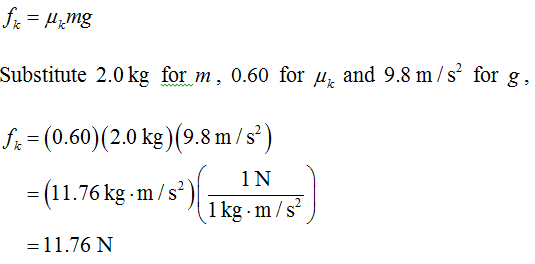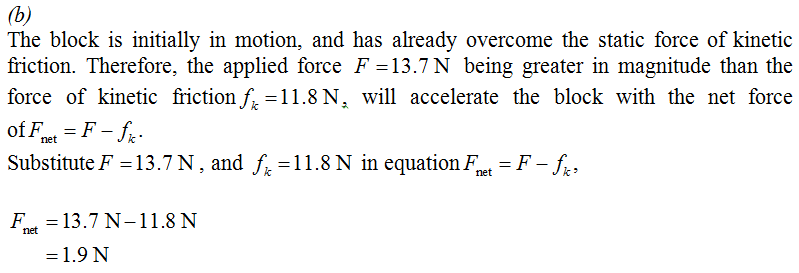×#### Thank you for registering.

One of our academic counsellors will contact you within 1 working day.

Click to Chat

1800-1023-196

+91-120-4616500

CART 0

• 0

MY CART (5)

Use Coupon: CART20 and get 20% off on all online Study Material

ITEM
DETAILS
MRP
DISCOUNT
FINAL PRICE
Total Price: Rs.

There are no items in this cart.
Continue Shopping
```
A 2.0-kg block of wood is on a level surface where μ s and μ k = 0.60. A 13.7-N force is being applied to the block parallel to the surface. (a) If the block was originally at rest, then (A) it will remain at rest, and the force of friction will be about 15.7 N. (B) it will remain at rest, and the force of friction will be about 13.7 N. (C) it will remain at rest, and the force of friction will be 11.8 N. (D) it will begin to slide with a net force of about 1.9 acting on the block. (b) If the block was originally in motion, and the 13.7-N applied force is in the direction of motion, then (A) it will accelerate under a net force of about 1.9 N. (B) it will move at constant speed. (C) it will decelerate under a net force of about 1.9 N. (D) it will decelerate under a net force of 11.8 N.
A 2.0-kg block of wood is on a level surface where μs and μk = 0.60. A 13.7-N force is being applied to the block parallel to the surface. (a) If the block was originally at rest, then(A) it will remain at rest, and the force of friction will be about 15.7 N.(B) it will remain at rest, and the force of friction will be about 13.7 N.(C) it will remain at rest, and the force of friction will be 11.8 N.(D) it will begin to slide with a net force of about 1.9 acting on the block.(b) If the block was originally in motion, and the 13.7-N applied force is in the direction of motion, then(A) it will accelerate under a net force of about 1.9 N.(B) it will move at constant speed.(C) it will decelerate under a net force of about 1.9 N.(D) it will decelerate under a net force of 11.8 N.

```
5 years ago

```							The correct option is:(a)(B) it will remain at rest, and the force of friction will be 13.7 N.(b)(A) it will accelerate under a net force of about 1.9 N.The magnitude of static force of friction on block is:fs = μsNHere, N is the normal force on the block.The block rest on a level surface, therefore the normal force should be equal to the weight of the block, that is, N = mg .Substitute the same in equationfs = μsN.fs = μsN.Here, m is the mass of the block, and g is the acceleration due to gravity.Round off to three significant figures,fs = 15.7 NSimilarly, the magnitude of force of kinetic friction is given as:Round off to three significant figures,fk = 11.8 N(a)It is important to note that the applied force F = 13.7 N is greater than the kinetic force of friction fk = 11.8 N, and is capable of moving the object. However the object moves when the applied force has taken over the rest state of the object appearing due to static force of friction.Therefore, to move the object, the applied force is initially larger than force of static friction, and once the motion has started, the block can be moved if the magnitude of applied force is greater than kinetic force of friction. From this, one can deduce that the applied force F = 13.7 N is insufficient in moving the object against static friction fs = 15.7 N, and cannot move the object.Therefore, the object remains at rest and experiences a static friction of magnitude equal to the magnitude of applied force, that is,13.7 N.Thus, (B) is the correct option, and the rest are ruled out.Therefore, the block will accelerate with a net force of 1.9 N.Thus, (A) is the correct option, and the rest are ruled out.
```
5 years ago
Think You Can Provide A Better Answer ?

## Other Related Questions on Mechanics

View all Questions »### Course Features

• 101 Video Lectures
• Revision Notes
• Previous Year Papers
• Mind Map
• Study Planner
• NCERT Solutions
• Discussion Forum
• Test paper with Video Solution### Course Features

• 110 Video Lectures
• Revision Notes
• Test paper with Video Solution
• Mind Map
• Study Planner
• NCERT Solutions
• Discussion Forum
• Previous Year Exam Questions